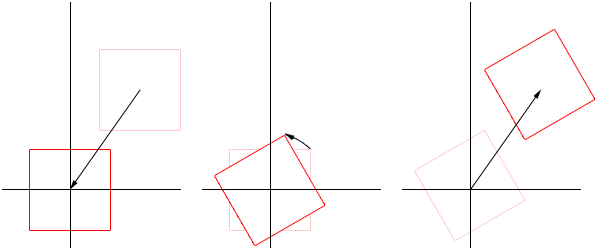# mpatric.com

## Extending Texture2D part 1 - rotation

The `Texture2D` class was included with Apple's CrashLanding iOS sample application. Many developers pulled it out of this sample app to use in their own apps as a quick and easy way of rendering 2D images of any size and a variety of colour formats in OpenGL. I find it useful, particularly when prototyping ideas, however the class is limited in its functionality. I developed a small set of extensions for `Texture2D` for my own purposes and thought others might find them useful. This post describes how to extend `Texture2D` to allow you to rotate it about its center by an arbitrary angle. The Objective-C code is available on github.The aim is to extend `Texture2D` to provide two new methods, where `rotationAngle` is the angle in degrees, counter-clockwise (or clockwise with a negative angle) to rotate the texture about its center:

``````- (void) drawInRect:(CGRect)rect rotatedBy:(float)rotationAngle;
- (void) drawAtPoint:(CGPoint)point rotatedBy:(float)rotationAngle;
``````

In order to do this, a new co-ordinate needs to be determined for each corner of the bounding rectangle of the `Texture2D` object. It's much easier to rotate points about the origin than about an arbitrary point, so the approach I take is to translate the bounding rectangle so that its center is at the origin, rotate the corners, then translate the result back so its center is back in its initial position.### Translating to the origin

To translate the bounding rectangle so that its center is on the origin, simply subtract the co-ordinates of the center from the co-ordinates of each of the corners.

To rotate each corner around the origin, we need a formula to rotate a point (or, if you prefer, a vector that starts at the origin) about the origin.Determining values for x' and y':

``````            x' = r cos(α')
Substitute: α' = α + θ
x' = r cos(α + θ)
x' = r(cos(α)cos(θ) - sin(α)sin(θ))
Substitute: cos(α) = x/r and sin(α) = y/r
x' = r(x/r cos(θ) - y/r sin(θ))
x' = x cos(θ) - y sin(θ)
``````

Similarly it can be shown that

``````            y' = x sin(θ) + y cos(θ)
``````

### Translating back

To translate the bounding rectangle of the `Texture2D` object back so that its center is in its initial position, simply add the initial co-ordinates of the center back to the co-ordinates of each of the corners.

### Bringing it all together

Bringing the all together, for each corner of the bounding rectangle of a `Texture2D` object:

``````Let cx, cy be the center point of the bounding rectangle
For each corner, with co-ordinate (x, y):
x' = cx + ((x - cx)cos(θ) - (y - cy)sin(θ))
y' = cy + ((x - cx)sin(θ) + (y - cy)cos(θ))
cx and cy are simply:
cx = (x + width)/2
cy = (y + height)/2
``````

### The code

``````#define ROTATE_ABOUT_POINT(x, y, cx, cy, cosTheta, sinTheta) CGPointMake(((((x) - (cx)) * (
cosTheta)) - (((y) - (cy)) * (sinTheta))) + (cx), ((((x) - (cx)) * (sinTheta)) + (((y) -
(cy)) * (cosTheta))) + (cy));

#define DEGREES_TO_RADIANS(__ANGLE__) ((__ANGLE__) / 180.0 * M_PI)

- (void) drawInRect:(CGRect)rect rotatedBy:(float)rotationAngle {
CGPoint center = CGPointMake(rect.origin.x + (rect.size.width / 2),
rect.origin.y + (rect.size.height / 2));
center.x, center.y,
cosTheta, sinTheta);
CGPoint p2 = ROTATE_ABOUT_POINT(rect.origin.x + rect.size.width, rect.origin.y,
center.x, center.y,
cosTheta, sinTheta);
CGPoint p3 = ROTATE_ABOUT_POINT(rect.origin.x, rect.origin.y + rect.size.height,
center.x, center.y,
cosTheta, sinTheta);
CGPoint p4 = ROTATE_ABOUT_POINT(rect.origin.x + rect.size.width,
rect.origin.y + rect.size.height,
center.x, center.y,
cosTheta, sinTheta);
GLfloat  coordinates[] = {
0, self.maxT,
self.maxS, self.maxT,
0, 0,
self.maxS, 0
};
GLfloat vertices[] = {
p1.x, p1.y, 0.0,
p2.x, p2.y, 0.0,
p3.x, p3.y, 0.0,
p4.x, p4.y, 0.0
};
glBindTexture(GL_TEXTURE_2D, self.name);
glVertexPointer(3, GL_FLOAT, 0, vertices);
glTexCoordPointer(2, GL_FLOAT, 0, coordinates);
glDrawArrays(GL_TRIANGLE_STRIP, 0, 4);
}

- (void) drawAtPoint:(CGPoint)point rotatedBy:(float)rotationAngle {
[self drawInRect:CGRectMake(point.x - self.imageSize.width / 2, point.y -
self.imageSize.height / 2, self.imageSize.width, self.imageSize.height)
rotatedBy: rotationAngle];
}
``````

I've used a macro to make the code a bit more readable and calculate cos(θ) and sin(θ) each once and pass them into the macro for performance reasons. Have a look at `MyTexture2D` class in the code on github for a full implementation of this. The project also includes a small iOS demonstration app showing it in action.Posted Fri 21 Oct 2011 by Michael Patricios

Tags: Development, Objective-C, OpenGL

•